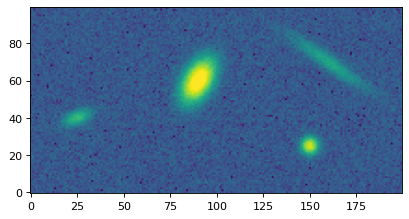# make_4gaussians_image¶

`photutils.datasets.``make_4gaussians_image`(noise=True)[source]

Make an example image containing four 2D Gaussians plus a constant background.

The background has a mean of 5.

If `noise` is `True`, then Gaussian noise with a mean of 0 and a standard deviation of 5 is added to the output image.

Parameters
noisebool, optional

Whether to include noise in the output image (default is `True`).

Returns
image

Image containing four 2D Gaussian sources.

Examples

```from photutils.datasets import make_4gaussians_image
image = make_4gaussians_image()
plt.imshow(image, origin='lower', interpolation='nearest')
```